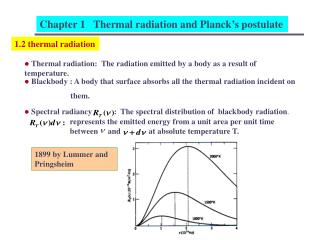DownloadDownload PresentationChapter 1 Thermal radiation and Planck’s postulate

# Chapter 1 Thermal radiation and Planck’s postulate

Download Presentation## Chapter 1 Thermal radiation and Planck’s postulate

- - - - - - - - - - - - - - - - - - - - - - - - - - - E N D - - - - - - - - - - - - - - - - - - - - - - - - - - -
##### Presentation Transcript

1. Chapter 1 Thermal radiation and Planck’s postulate 1.2 thermal radiation • Thermal radiation: The radiation emitted by a body as a result of temperature. • Blackbody : A body that surface absorbs all the thermal radiation incident on them. • Spectral radiancy : The spectral distribution of blackbody radiation. represents the emitted energy from a unit area per unit time between and at absolute temperature T. 1899 by Lummer and Pringsheim

2. Chapter 1 Thermal radiation and Planck’s postulate • The spectral radiancy of blackbody radiation shows that: • (1) little power radiation at very low frequency • (2) the power radiation increases rapidly as ν increases from very • small value. • (3) the power radiation is most intense at certain for particular • temperature. • drops slowly, but continuously as ν increases • , and • increases linearly with increasing temperature. • (6) the total radiation for all ν ( radiancy ) • increases less rapidly than linearly with increasing temperature.

3. Chapter 1 Thermal radiation and Planck’s postulate • Stefan’s law (1879): Stefan-Boltzmann constant • Wien’s displacement (1894): 1.3 Classical theory of cavity radiation • Rayleigh and Jeans (1900): (1) standing wave with nodes at the metallic surface (2) geometrical arguments count the number of standing waves (3) average total energy depends only on the temperature • one-dimensional cavity: one-dimensional electromagnetic standing wave

4. Chapter 1 Thermal radiation and Planck’s postulate • for all time t, nodes at standing wave the number of allowed standing wave between ν and ν+dν two polarization states

5. Chapter 1 Thermal radiation and Planck’s postulate • for three-dimensional cavity the volume of concentric shell The number of allowed electromagnetic standing wave in 3D Proof: propagation direction λ/2 nodal planes λ/2

6. Chapter 1 Thermal radiation and Planck’s postulate • for nodes: considering two polarization state Density of states per unit volume per unit frequency

7. Chapter 1 Thermal radiation and Planck’s postulate • the law of equipartition energy: For a system of gas molecules in thermal equilibrium at temperature T, the average kinetic energy of a molecules per degree of freedom is kT/2, is Boltzmann constant. • average total energy of each standing wave : • the energy density between ν and ν+dν: Rayleigh-Jeans blackbody radiation ultravioletcatastrophe

8. Chapter 1 Thermal radiation and Planck’s postulate 1.4 Planck’s theory of cavity radiation • Planck’s assumption: and • the origin of equipartition of energy: Boltzmann distribution probability of finding a system with energy between ε and ε+dε

9. Chapter 1 Thermal radiation and Planck’s postulate • Planck’s assumption: (1) small ν (2) large large ν Planck constant Using Planck’s discrete energy to find

10. Chapter 1 Thermal radiation and Planck’s postulate

11. Chapter 1 Thermal radiation and Planck’s postulate • energy density between ν and ν+dν: Ex: Show solid angle expanded by dA is spectral radiancy:

12. Chapter 1 Thermal radiation and Planck’s postulate Ex: Use the relation between spectral radiancy and energy density, together with Planck’s radiation law, to derive Stefan’s law

13. Chapter 1 Thermal radiation and Planck’s postulate Ex: Show that Set by consecutive partial integration Fourier series expansion

14. Chapter 1 Thermal radiation and Planck’s postulate Ex: Derive the Wien displacement law ( ), Solve by plotting: find the intersection point for two functions Y intersection points: X

15. Chapter 1 Thermal radiation and Planck’s postulate 1.5 The use of Planck’s radiation law in thermometry optical pyrometer (1) For monochromatic radiation of wave length λ the ratio of the spectral intensities emitted by sources at and is given by standard temperature ( Au ) unknown temperature (2) blackbody radiation supports the big-bang theory.

16. Chapter 1 Thermal radiation and Planck’s postulate 1.6 Planck’s Postulate and its implication Planck’s postulate: Any physical entity with one degree of freedom whose “coordinate” is a sinusoidal function of time (i.e., simple harmonic oscillation can posses only total energy Ex: Find the discrete energy for a pendulum of mass 0.01 Kg suspended by a string 0.01 m in length and extreme position at an angle 0.1 rad. The discreteness in the energy is not so valid.